Question

# consider that an induction motor is connected to a 3 phase source and the motor is...

consider that an induction motor is connected to a 3 phase source and the motor is rotating in a particular direction. if the connections at the two terminals of the motor are interchanged, what will be the effect of the motor?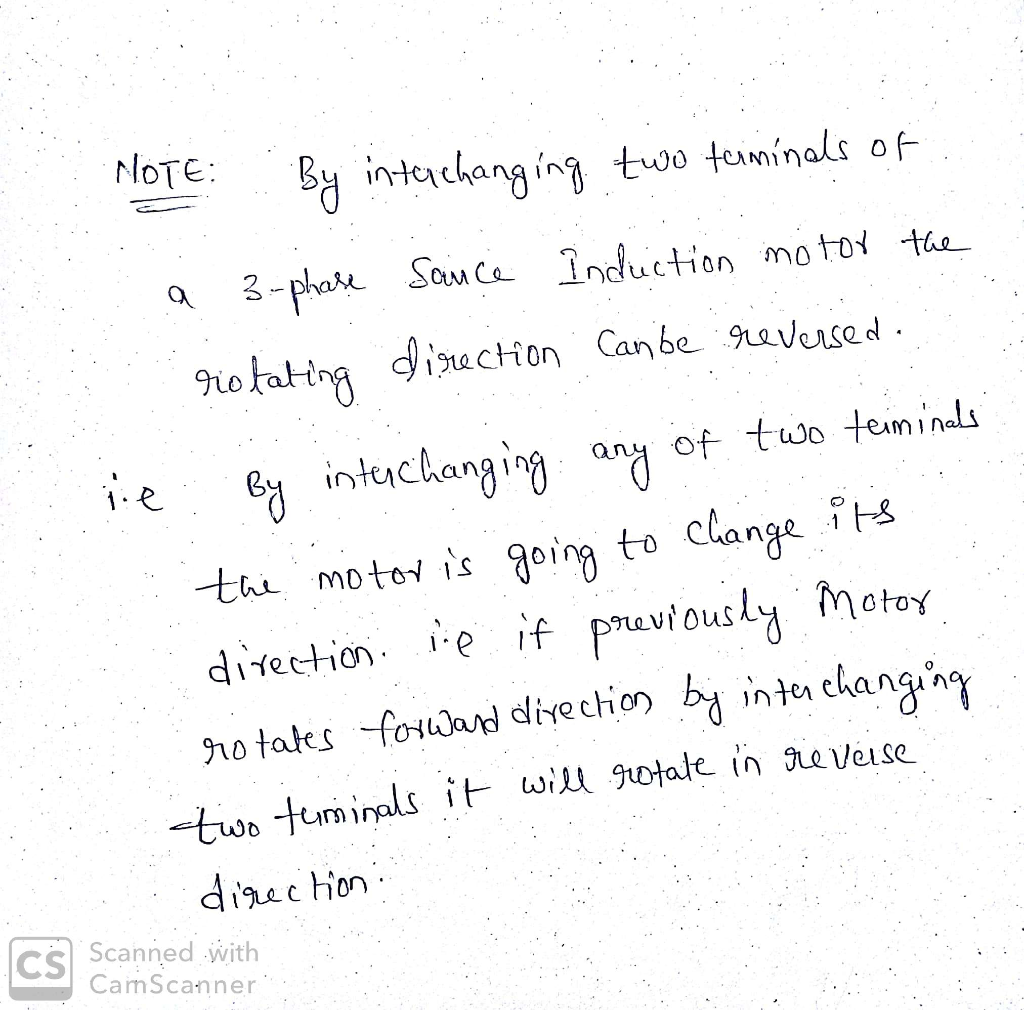#### Earn Coins

Coins can be redeemed for fabulous gifts.

Similar Homework Help Questions
• ### Consider a 50 kW, 3-phase, 380 V line-to-line, 50 Hz, 6 pole Y-connected wound-rotor induction motor....

Consider a 50 kW, 3-phase, 380 V line-to-line, 50 Hz, 6 pole Y-connected wound-rotor induction motor. The stator winding ac resistance is 0.1 Ω/phase. The effective stator-to-rotor turns ratio is 2. The exciting branch is negligible. It is found that when an external resistor of 0.09 Ω/phase is connected to the rotor terminals maximum starting torque of 1150 Nm is obtained. a. Compute the internal mechanical power and the internal torque developed by this motor when it drives a load...

• ### Problem 3: Consider an 6 pole 3-phase AC induction motor being driven at 60 Hz a)...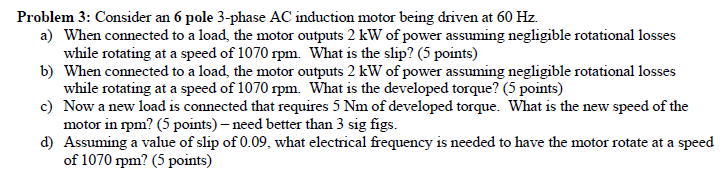Problem 3: Consider an 6 pole 3-phase AC induction motor being driven at 60 Hz a) b) c) d) When connected to a load, the motor outputs 2 kW of power assuming negligible rotational losses while rotating at a speed of 1070 rpm. What is the slip? (5 points) When connected to a load, the motor outputs 2 kW of power assuming negligible rotational losses while rotating at a speed of 1070 rpm. What is the developed torque? (5 points)...

• ### The equivalent circuit of a 440 V, 3-phase, 8 pole, and 50 Hz star-connected induction motor (HELP)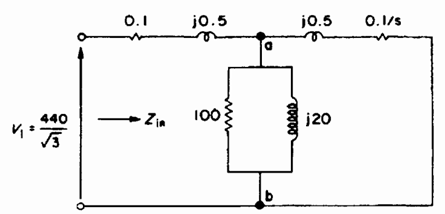Question:  The equivalent circuit of a 440 V, 3-phase, 8 pole, and 50 Hz star-connected induction motor is shown in figure 1. The short-circuit test is conducted with a locked rotor and a line current of 80 A. The open-circuit test is conducted by supplying the stator winding at rated voltage and at the rotor is rotating freely without any mechanical load.           Fig. 1 The per-phase equivalent circuit of a 3-phase induction motora)  Determine the line voltage and power factor on the...

• ### Prelab 1. In your lab notebook draw the schematic diagram for a three-phase wye-connected induction motor...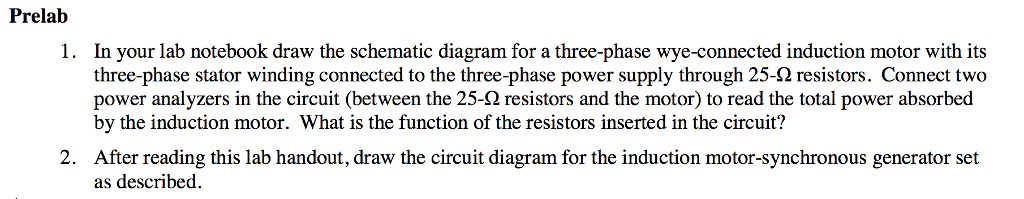Prelab 1. In your lab notebook draw the schematic diagram for a three-phase wye-connected induction motor with its three-phase stator winding connected to the three-phase power supply through 25-Ω resistors. Connect two er analyzers in the circuit (between the 25-Ω resistors and the motor) to read the total power absorbed by the induction motor. What is the function of the resistors inserted in the circuit? 2. After reading this lab handout, draw the circuit diagram for the induction motor-synchronous generator...

• ### Three-phase induction motor The three-phase induction motor nameplate gives the following informa...

Three-phase induction motor The three-phase induction motor nameplate gives the following information: 100 HP, 6 poles, 600 V, 60 Hz, PF =0.87. Rated motor slip s=0.0275 and rated motor current is 91.7 A. Measured at rated speed friction and ventilation losses are 1100 W. Measured between two motor terminals resistance is 0.305 Ω. f) For a load such where the motor rotates at the speed of 1180 r / min, determine shaft torque and calculate the mechanical power in HP,...

• ### 4. Consider a stepper motor with two phases. Phase 1 is wound with two identical coils. Phase 2 i...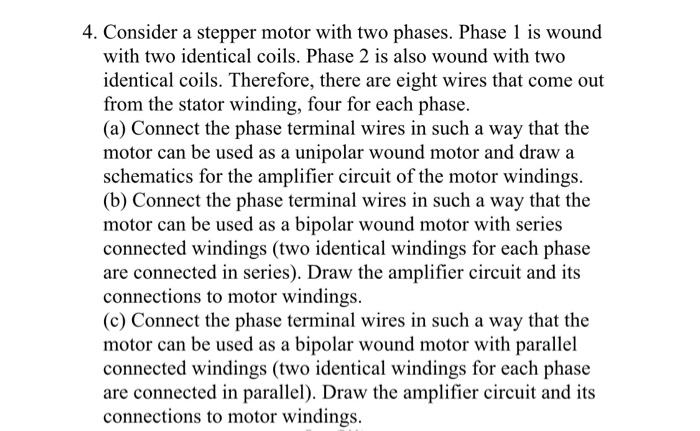4. Consider a stepper motor with two phases. Phase 1 is wound with two identical coils. Phase 2 is also wound with two identical coils. Therefore, there are eight wires that come out from the stator winding, four for each phase (a) Connect the phase terminal wires in such a way that the motor can be used as a unipolar wound motor and draw:a schematics for the amplifier circuit of the motor windings (b) Connect the phase terminal wires in...

• ### 6.12 A three-phase, Y-connected, 460-V (line-line), 37-kW, 60-Hz, four-pole induction motor has the following equivalent-circuit parameters...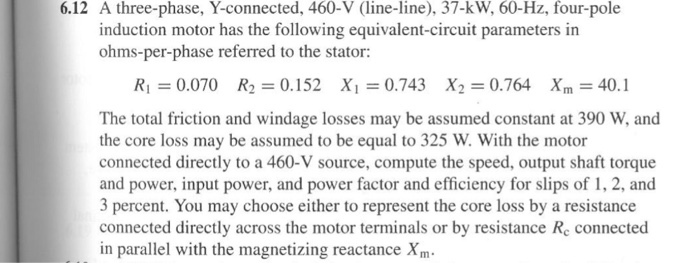6.12 A three-phase, Y-connected, 460-V (line-line), 37-kW, 60-Hz, four-pole induction motor has the following equivalent-circuit parameters in ohms-per-phase referred to the statr: R10.070 R2 0.152 X 0.743 X2 0.764 Xm 40.1 The total friction and windage losses may be assumed constant at 390 W, and the core loss may be assumed to be equal to 325 W. With the motor connected directly to a 460-V source, compute the speed, output shaft torque and power, input power, and power factor and...

• ### 44. The direction of rotation of an induction motor depends on a. the frequency of the...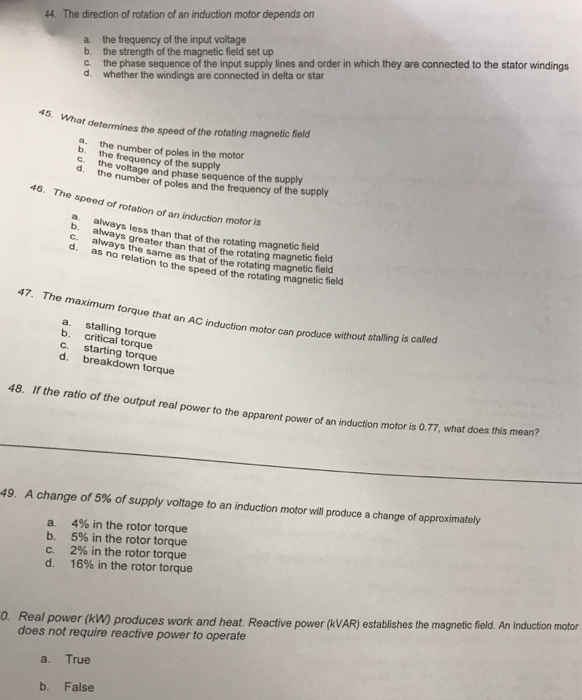44. The direction of rotation of an induction motor depends on a. the frequency of the input voltage b. the strength of the magnetic field set up c. the phase sequence of the input supply lines and order in which they are connected to the stator windings d. whether the windings are connected in delta or star determines the speed of the rotating magnetic field a. the number of poles in the motor b. the frequency of the supply d...

• ### Equivalent circuit of 440V, 3-phase, 8 pole, 50 Hz star-connected induction motorThe equivalent circuit of a 440 V, 3-phase, 8 pole, and 50 Hz star-connected induction motor is shown in figure 1. The short-circuit test is conducted with a locked rotor and a line current of 80 A. The open-circuit test is conducted by supplying the stator winding at rated voltage and at the rotor is rotating freely without any mechanical load.a)     Determine the line voltage and power factor on the short circuit test;b)   We need to apply a low...